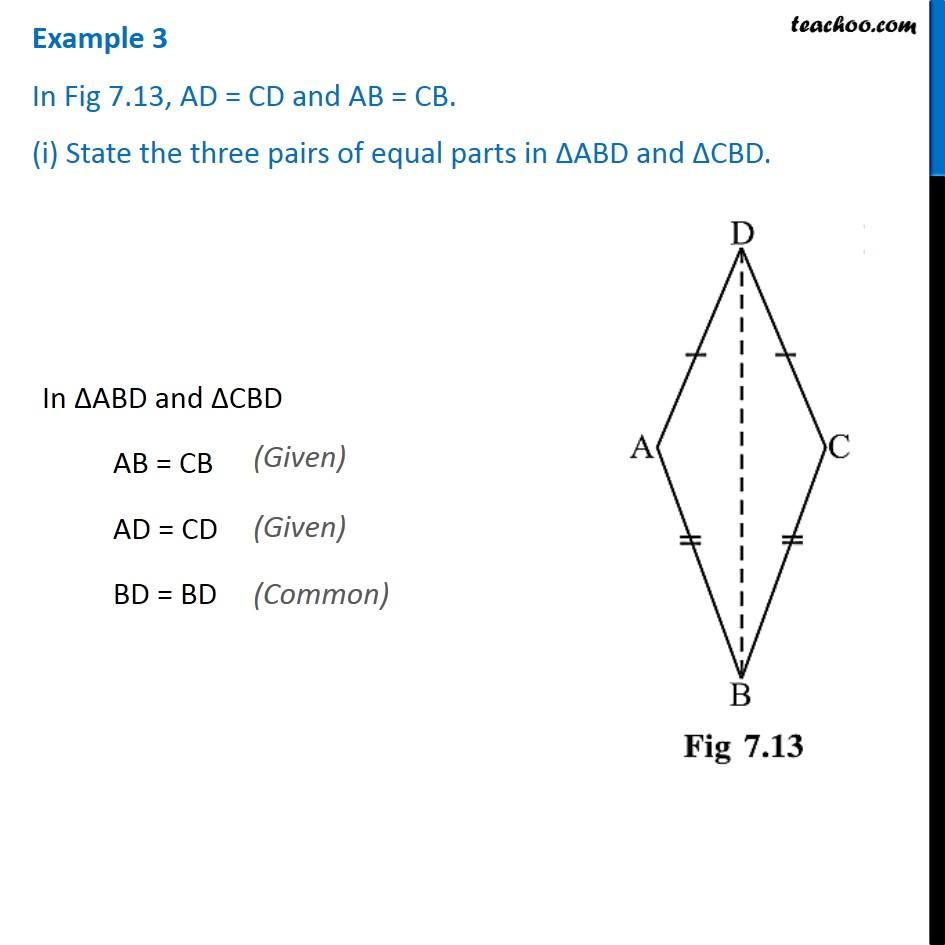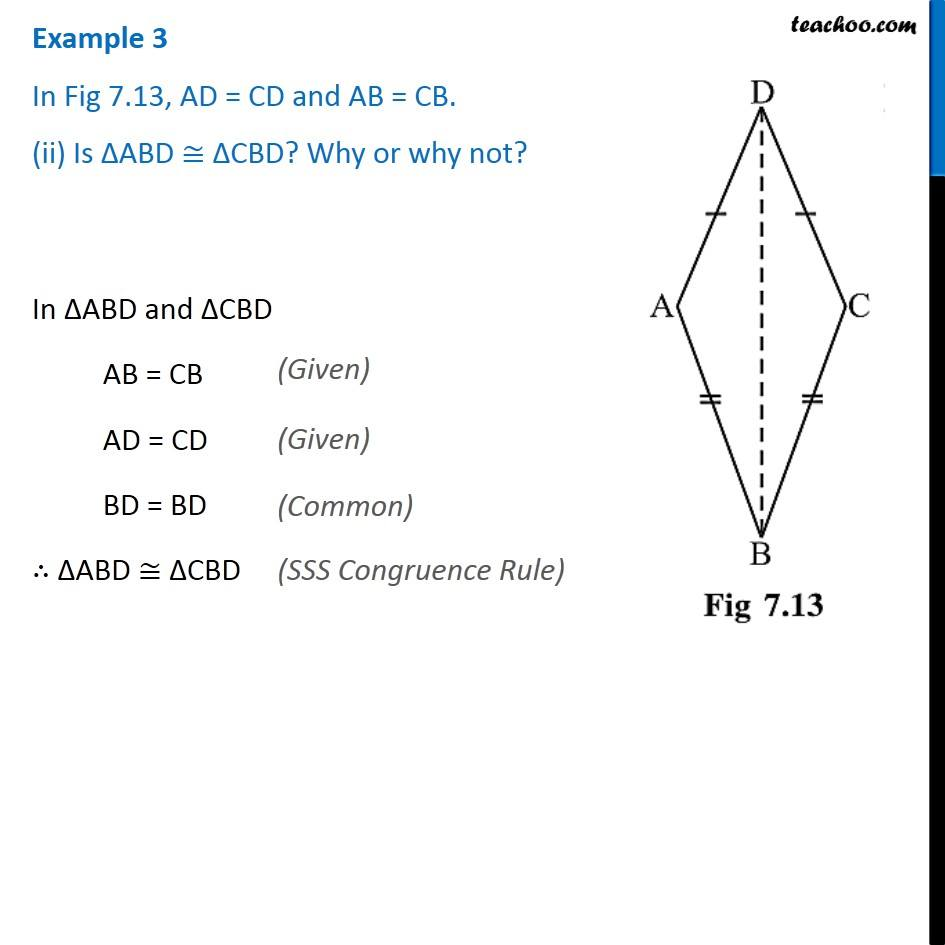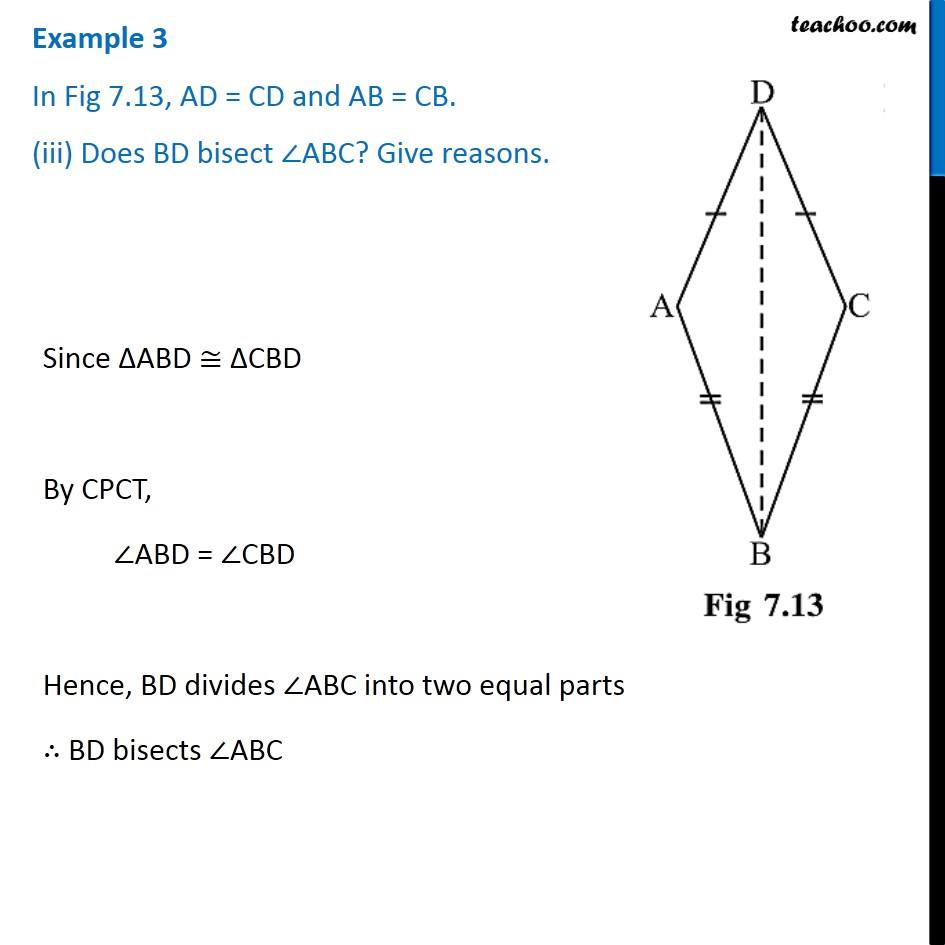SSS Congruency Criteria

Congruence of Triangles
Concept wiseLearn in your speed, with individual attention - Teachoo Maths 1-on-1 Class

### Transcript

Example 3 - Chapter 7 Class 7 NCERT In Fig 7.13, AD = CD and AB = CB. (i) State the three pairs of equal parts in ∆ABD and ∆CBD. In ∆ABD and ∆CBD AB = CB (Given) AD = CD (Given) BD = BD (Common) Example 3 In Fig 7.13, AD = CD and AB = CB. (ii) Is ∆ABD ≅ ∆CBD? Why or why not? In ∆ABD and ∆CBD AB = CB (Given) AD = CD (Given) BD = BD (Common) ∴ ∆ABD ≅ ∆CBD (SSS Congruence Rule) Example 3 In Fig 7.13, AD = CD and AB = CB. (iii) Does BD bisect ∠ABC? Give reasons. Since ∆ABD ≅ ∆CBD By CPCT, ∠ABD = ∠CBD Hence, BD divides ∠ABC into two equal parts ∴ BD bisects ∠ABC